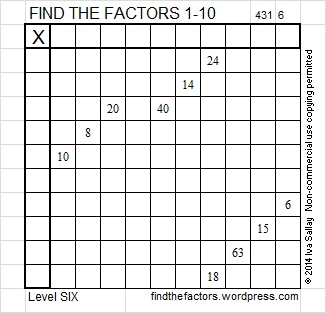# 198 and Level 6

• 198 is a composite number.
• Prime factorization: 198 = 2 × 3 × 3 × 11, which can be written 198 = 2 × 3² × 11
• The exponents in the prime factorization are 1, 2, and 1. Adding one to each and multiplying we get (1 + 1)(2 + 1)(1 + 1) = 2 × 3 × 2 = 12. Therefore 198 has exactly 12 factors.
• Factors of 198: 1, 2, 3, 6, 9, 11, 18, 22, 33, 66, 99, 198
• Factor pairs: 198 = 1 × 198, 2 × 99, 3 × 66, 6 × 33, 9 × 22, or 11 × 18
• Taking the factor pair with the largest square number factor, we get √198 = (√9)(√22) = 3√22 ≈ 14.0712Since 1 + 9 + 8 = 18, a multiple of 9, 198 is divisible by 9. Here is one of several possible factor trees for 198:Excel file of puzzles and previous week’s factor solutions: 10 Factors 2014-08-04

The common factors of 20 and 40 are 4, 5, and 10. Since 14 and either 8, 10, or 6 will use both 2’s, 10 cannot be the CF. If we choose 4 as the common factor, then 5 and 10 would be placed in the top row followed by 3, the factor of 15. However, then we wouldn’t be able to use either 3 or 6 as the common factor of 24 and 18 because 3 would already be in the top row and 4 would already be in the 1st column.  Therefore, the common factor of 20 and 40 has to be 5.This site uses Akismet to reduce spam. Learn how your comment data is processed.